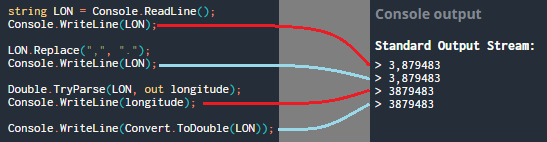# Defribillators puzzle discussion

#269

Why / 200.0 and *1000?

#270

Hi guys,
little issue with this puzzle, when I am using ‘pi’ and ‘cos’ as requested it tells me it doesn’t exist…despite the “math import” at the beginning…
Thanks in advance

#271

In Python?
How is the import written?

#272

Yeah sorry, it’s in Python (not Python 3), and it’s written “import math”… I tried several time to refresh, didn’t change anything

#273

Are you using math.cos?

#274

No I didn’t try… should I you math.pi too then ?

#275

Great it worked ! thank you !
I never had to use this way, does it work like this for every package ?

#276

Yes, you can do, either:

``````import math
print(math.cos(math.pi))
``````

Or:

``````from math import cos,pi
print(cos(pi))``````

#277

Okay thank you for the explanation
So for example I could write my code normally and at the end, go back to the top of the code and do “from math import cos,pi” and it should work right ?
Thank you again guys !

#278

You should write from math import cos,pi immediately even if you can write it everywhere in your code (before the use of cos and pi of course).

#279

Hi! In C++, Like a lot of people here, I have complete all tests but I don’t have 100%.
For me, the problem is in the first test. I don’t know if the name is important but during the game the first test is “Example” and in the score page it is “Single possibility”.
I have used double or float, doesn’t matter. I have put << endl for the answer.
I don’t have other idea.
Thx

#280

Does your code work if the information of only 1 defibrillator is provided in the game input?

#281

To be sure, I tested to put “N = 1” before the loop to simulate only 1 defibrillator. It works.

#282

Chingmann has helped me and it works. I forgot to convert degree to radian#283

My C# code, which works fine in VS, isn’t returning the expected results on here.
After finnicking about for longer than I’d like to admit it turned out my numbers were off, though I beleive that I converted them correctly.
Am I being an Idiot?#284

Do you see that your second Console.WriteLine is the same as the first one? That means you have to fix the line between them.

#285

Hint: string.Replace() does not act the way you think it does…

#286

I already figured it out thanks to Chingmann. It was even more glaringly obvious in hindsight than usual and I’m stricken with shame. But such boorishly blatant blunders bare benefits, I will not make this mistake again.

#287

I also had the problem, that the formula provided by the webpage listed in external resources is too precise!
Simple trig will do the deal and get you 100%

Here is the javascript:
function calculateDistance(user, defibrillator){
let distance = Math.sqrt(Math.pow(defibrillator.latitude - user.latitude, 2) + Math.pow(defibrillator.longitude - user.longitude, 2));
return distance;
}

#289

Hi,

I have a problem with my code.

My code is working only in 50% of cases.

Somebody can understand the reason?

JAVA

``````import java.util.*;
import java.io.*;
import java.math.*;
import java.text.*;

class Solution {
public static void main(String args[]) {
Scanner in = new Scanner(System.in);
double lou = Double.parseDouble(in.next().replaceAll("," , "."));
//System.out.println(lou);
double lau = Double.parseDouble(in.next().replaceAll("," , "."));
int n = in.nextInt();
if (in.hasNextLine()) {
in.nextLine();
}
int[] id = new int[n];
String[] nam = new String[n];
String[] add = new String[n];
String[] pho = new String[n];
double[] lon = new double[n];
double[] lat = new double[n];

for (int i = 0; i < n; i++) {
char[] def = in.nextLine().toCharArray();
int dle = def.length;
int con = 0;
String aux = "";
for(int j = 0; j < dle; j++){

if(def[j] != ';'){
aux += def[j];
con--;

}
else if(con == 0){
id[i] = Integer.parseInt(aux);
aux = "";
}
else if(con == 1){
nam[i] = aux;
aux = "";
}
else if(con == 2){
add[i] = aux;
aux = "";
}
else if(con == 3){
pho[i] = aux;
aux = "";
}
else if(con == 4){
aux = aux.replaceAll("," , ".");
//System.out.println(aux);
lon[i] = Double.parseDouble(aux);
}
else if(con == 5){
aux = aux.replaceAll("," , ".");
lat[i] = Double.parseDouble(aux);
}
con++;
//System.out.println(con);
}
}
double[] dis = new double[n];
int mai = 0;
for(int i = 0; i < n; i++){
double x = (lon[i] - lou) * Math.cos((lat[i] + lau) / 2);
double y = lat[i] - lau;
double res = (Math.sqrt(x * x + y * y) * 6371);
//System.out.println(res);
dis[i] = res;
if(dis[i] <= dis[mai]){
mai = i;
//System.out.println(dis[i]);
}
//System.out.println(i);
}
System.out.print(nam[mai]);

}
}
``````

Call me in private.